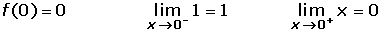If a function is differentiable at point x = a, then the function is continuous at x = a.

The reciprocal may not be true, that is to say, there are functions that are continuous at a point which, however, may not be differentiable.

Study the continuity and differentiability of the following functions:First, study the continuity at x = 0.The function is not continuous, therefore it is not differentiable.First, study the continuity at x = 0.This function is continuous, so the differentiability can be studied.It is not differentiable at x = 0.f(x) = x² at x = 0.

The function is continuous at x = 0, so the differentiability can be studied.At x = 0, the function is continuous and differentiable.Did you like the article?(1 votes, average: 5.00 out of 5)Loading...

Emma

I am passionate about travelling and currently live and work in Paris. I like to spend my time reading, gardening, running, learning languages and exploring new places.

Did you like
this resource?

Bravo!